# Electric Field Formula

Electric Field Formula

Objects with electric charge emit electric fields. This electric field is the source of the electrostatic force that nearby charged objects experience. The electric field is a vector quantity, and the direction of the field lines depends on the sign of the source charge. Electric field vectors point away from positively charged sources, and toward negatively charged sources. The formula for the electric field includes the Coulomb constant, which is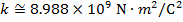. The Coulomb constant can also be written in terms of the permittivity of free space,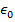. In that form, the Coulomb constant is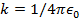. The unit of the electric field magnitude is Newtons per Coulomb, N/C.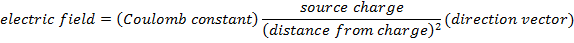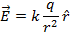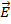= electric field vector at a certain position in space (N/C)

k = Coulomb constant (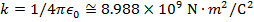)

q = charge of a single point source of the electric field (C)

r = distance from the source charge (m)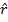= unit vector (length is 1), the direction of the electric field (unitless)

Electric Field Formula Questions:

1) Electric charges are often expressed as multiples of the smallest possible charge,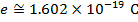. If a particle has a charge of +6e, what is the magnitude and direction of the electric field 1.000 mm away from the charge?

Answer: The direction of electric field vectors depend on the sign of the charge. The charge has a positive value, and so the direction of the electric field points away from the source. The first step to solving for the magnitude of the electric field is to convert the distance from the charge to meters:

r = 1.000 mmr = 0.001000 m

The magnitude of the electric field can be found using the formula: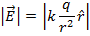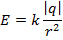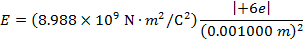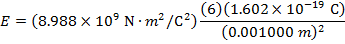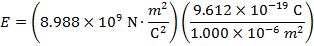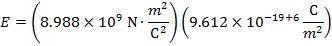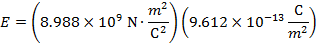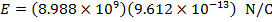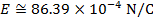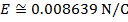The electric field 1.000 mm from the point charge has a magnitude of 0.008639 N/C, and is directed away from the charge.

2) A small metal ball has a charge of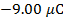(micro-Coulombs). Assuming the ball is a point source, what is the magnitude and direction of the electric field away from the source?

Answer: The direction of electric field vectors depend on the sign of the charge. The charge has a negative value, and so the direction of the electric field points toward the source. The first step to solving for the magnitude of the electric field is to convert the charge to Coulombs. The prefix "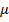", meaning "micro", indicates that the number is scaled by 10-6, and so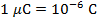. The charge of the source is: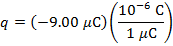The magnitude of the electric field can be found using the formula: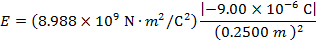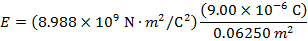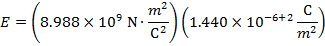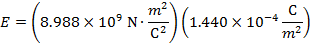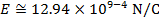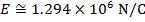The electric field 0.2500 m away from the small metal ball has a magnitude of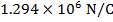, and is directed toward the charge.